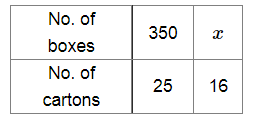# 350 boxes can be placed in 25 cartons.

Question:

350 boxes can be placed in 25 cartons. How many boxes can be placed in 16 cartons?

Solution:

Let x be the required number of boxes.Less number of boxes will require less number of cartons.

So, it is a case of direct proportion.

Now, $\frac{350}{25}=\frac{x}{16}$

$\Rightarrow x=\frac{350 \times 16}{25}$

$\Rightarrow x=224$

∴ 224 boxes can be placed in 16 cartoons.